# NNDL-1：使用神经网络识别手写数字

• 理解核心理念
• 而非围绕特定的程序库

## 使用神经网络识别手写数字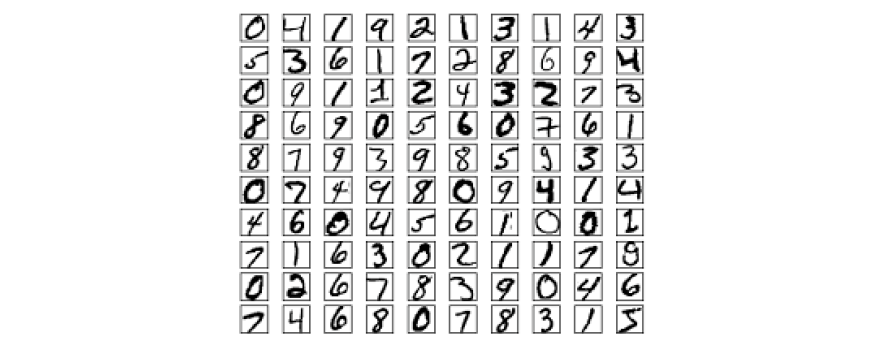• 具有挑战性：不同于传统建模
• 不需要复杂的算法和运算。

### 感知器 （Perceptron）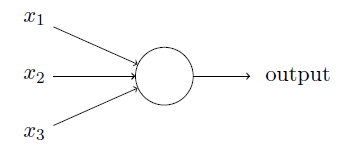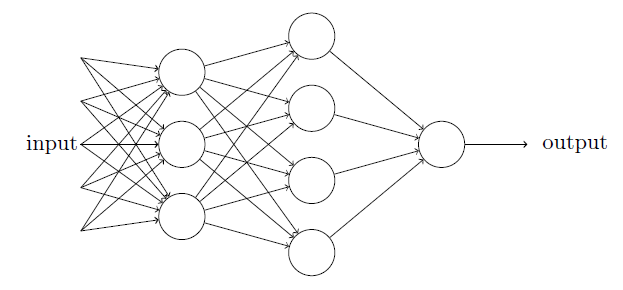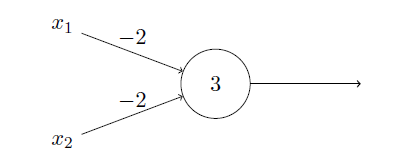### S型神经元（Sigmoid neurons）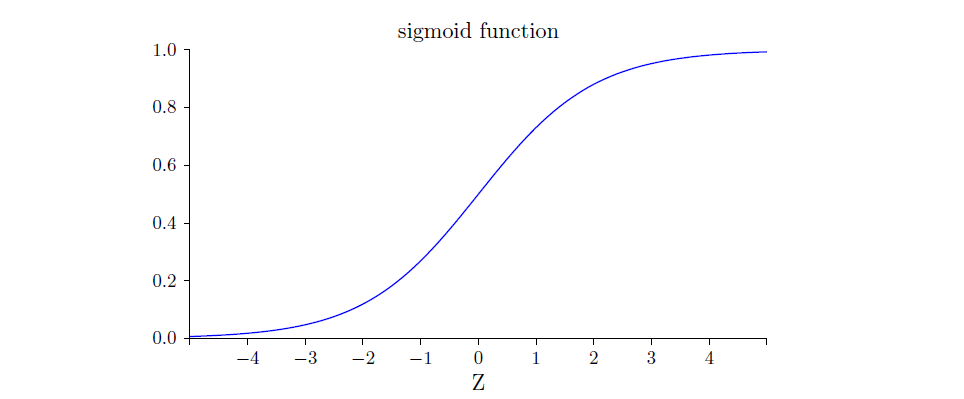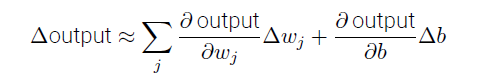• 证明所有权重和偏置乘以正常数c时，网络的行为不变。

从导数角度思考，最后的结果是导数最大值乘以了c，但是偶函数性质不变。

• 证明在假设$w\cdot x+b\neq0, \forall x$成立时，在正常量$c\rightarrow \infty$情况下，S型神经元的行为和感知器网络完全一致。

当$w\cdot x+b\neq0$时，我们可以保证S型神经元在z输出为0上下，能够保证唯一输出，而且根据上一问，c无穷大，导数最大值为无穷大，刚好时阶跃函数的导数：冲激函数；要是假设不满足，S型神经元可以保证$z=0$时输出为0.5，而感知器输出就是1了（按照规定）。

### 神经网络的架构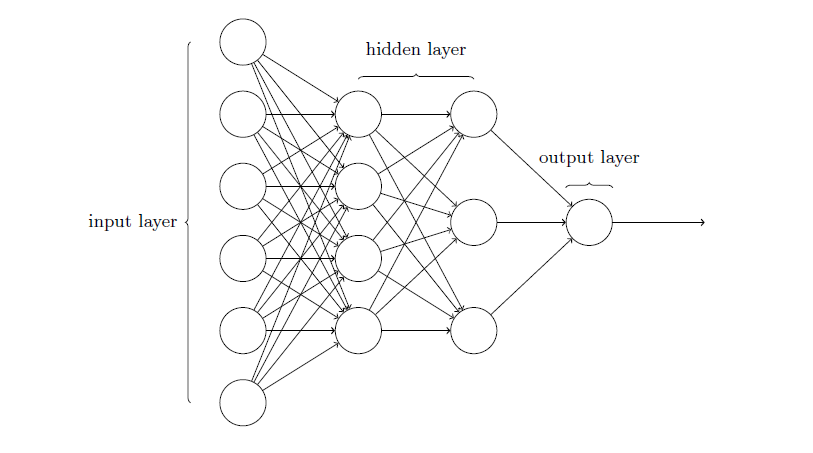输入层-隐藏层-输出层，历史上称为多层感知器（MLP）。

### 简单的手写数字分类网络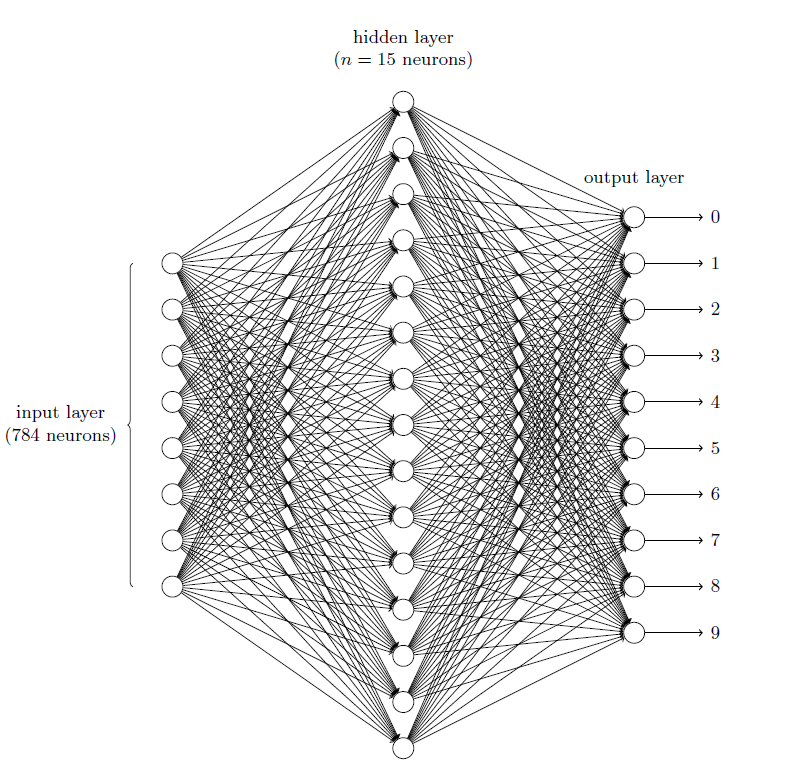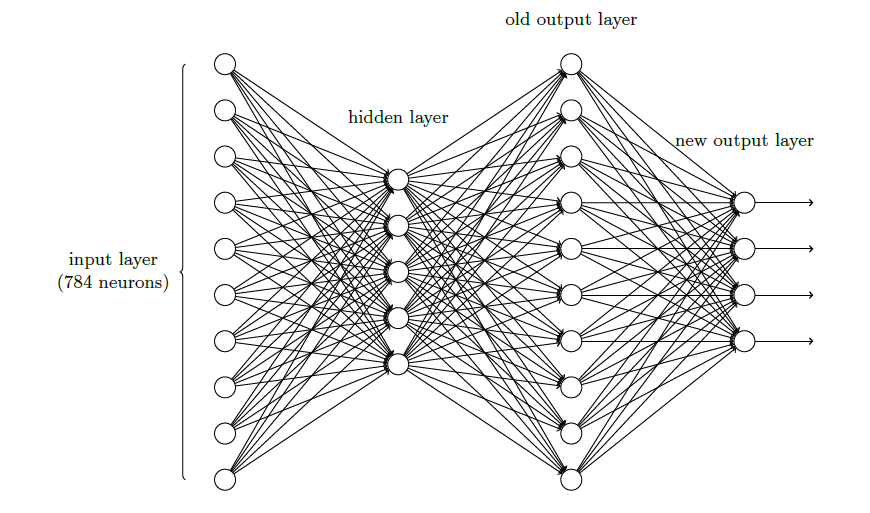### 使用梯度下降算法进行学习

MNIST数据分为训练集：60000张$28\times 28$图像，测试集：10000张$28\times 28$图像。网络输入$x: 28\times 28=784$的向量，输出$y(x)$：10维列向量。

代价函数（损失/目标）： $$C(w,b)\equiv\frac{1}{2n}\sum_x|y(x)-a|^2$$ $C$为二次代价函数，也叫均方误差或者MSE。为什么用二次？相对平滑，容易利用微小改变来最小化代价。为什么不用其他平滑的？之后再说

#### 数学基础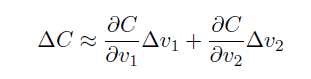• 证明取得最小值条件。

采用可惜-施瓦茨不等式，两个向量内积取最值当然是线性相关的时候。

• 如果代价函数时一元函数呢？如何用几何解释？

此时假设自变量为x，那么x的变化就是步长，视作定值，找最小值的过程简化为$C$从出发点开始减小到最近的极小值的过程。

#### 运用梯度下降

$$w_k \gets w_k-\eta\frac{\partial C}{\partial w_k}\\ b_l \gets b_l-\eta\frac{\partial C}{\partial b_l}$$

SGD每次随机选取的训练样本可以标记为$X_1, X_2,...,X_m$，叫做小批量数据（mini-batch）。假设m足够大，期望这个批数据的平均梯度应该大致等于全体样本的平均梯度。所以可以用mini-batch的梯度来估算整体梯度。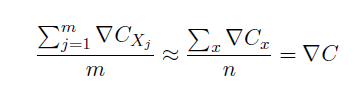⼈们有时候忽略1/n，直接取单个训练样本的代价总和，⽽不是取平均值。这对我们不能提前知道训练数据数量的情况下特别有效。例如，这可能发⽣在有更多的训练数据是实时产⽣的情况下。同样，⼩批量数据的更新规则有时也会舍弃前⾯的1/m。

SGD中每一个迭代期（epoch）进行一次参数更新：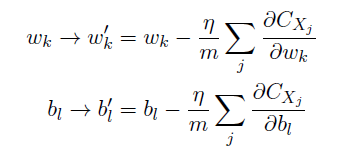• 优：计算消耗小；
• 缺：可能难以收敛，因为单个样本的代价梯度不容易用来估算整体梯度。

### 实现网络

核心代码规定了这个神经网络的特性，即神经元层数，层数的分配，每一层的神经元个数，权重，偏置。

 1 2 3 4 5 6 7 8 9  class Network: def __init__(self, sizes): """ sizes: [2, 3, 1] -> 2 neurons in input layer, 3 neurons in hidden layer, 1 neuron in output layer """ self.num_layers = len(sizes) self.sizes = sizes self.weights = [np.random.randn(j, i) for i, j in zip(self.sizes[:-1], self.sizes[1:])] self.biases = [np.random.randn(j, 1) for j in self.sizes[1:]] 

 1 2 3 4   def forward(self, a): for weight, bias in zip(self.weights, self.biases): a = sigmoid(np.dot(weight, a) + bias) return a 

  1 2 3 4 5 6 7 8 9 10 11 12 13 14 15 16 17 18 19 20 21 22 23 24 25 26 27 28 29 30 31 32   def SGD(self, training_data, epochs, mini_batch_size, learning_rate, test_data=None): if test_data: n_test = len(test_data) n = len(training_data) for t in range(epochs): random.shuffle(training_data) mini_batches = [training_data[k: k + mini_batch_size] for k in range(0, n, mini_batch_size)] for mini_batch in mini_batches: # Update parameters self.update_mini_batch(mini_batch, learning_rate) if test_data: print("Epoch {}: {} / {}".format(t, self.evaluate(test_data), n_test)) else: print("Epoch {} complete".format(t)) def update_mini_batch(self, mini_batch, learning_rate): """Update the network's weights and biases by applying gradient descent using backpropagation to a single mini batch. The "mini_batch" is a list of tuples "(x, y)", and "eta" is the learning rate. """ # Store the sum of all samples' nablas nabla_b = [np.zeros(b.shape) for b in self.biases] nabla_w = [np.zeros(w.shape) for w in self.weights] for x, y in mini_batch: sample_nabla_b, sample_nabla_w = self.backprop(x, y) # Sum update nabla_b = [nb + snb for nb, snb in zip(nabla_b, sample_nabla_b)] nabla_w = [nw + snw for nw, snw in zip(nabla_w, sample_nabla_w)] self.weights = [w - learning_rate / len(mini_batch) * nw for w, nw in zip(self.weights, nabla_w)] self.biases = [b - learning_rate / len(mini_batch) * nb for b, nb in zip(self.biases, nabla_b)] 

 1 2 3 4 5  >>> import mnist_loader >>> training_data, validation_data, test_data = mnist_loader.load_data_wrapper() >>> import network >>> net = network.Network([784, 30, 10]) >>> net.SGD(training_data, 30, 10, 3.0, test_data=test_data) # 30次迭代期，批数据大小为10，学习速率3.0 

 1 2 3 4 5 6 7  Epoch 0: 7631 / 10000 Epoch 1: 8440 / 10000 Epoch 2: 8564 / 10000 ... Epoch 27: 8882 / 10000 Epoch 28: 9153 / 10000 Epoch 29: 9140 / 10000 

### 效果反思

• 学习效率低了还是高了？
• 是否用了很差的初始权重或偏置？
• 训练数据是不是不够？
• 迭代期是否不够？
• 这种结构的神经网络，用来做这个任务是否可能？
• ...

 1 2 3 4  Epoch 27: 6494 / 10000 Epoch 28: 6959 / 10000 Epoch 29: 7019 / 10000 Training completed in 23 seconds 

first commit